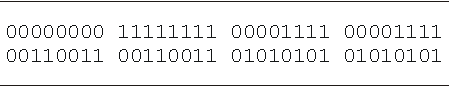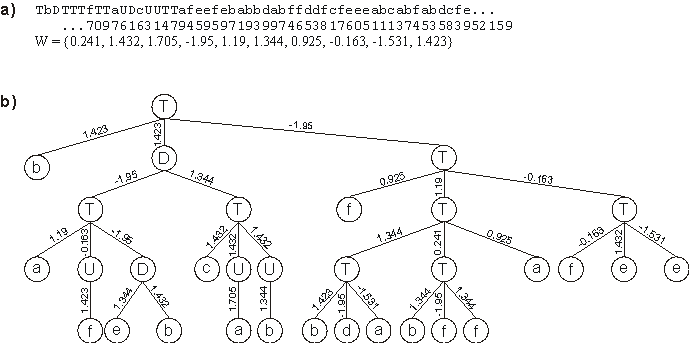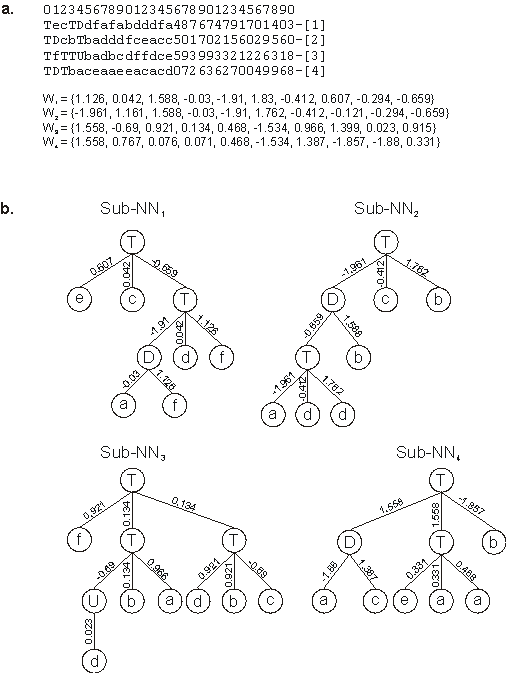Home
News
Author
Q&A
Tutorials
GEP Biblio
Contacts

Visit Gepsoft

Gene Expression Programming: Mathematical Modeling by an Artificial Intelligence

Neural network for the 6-multiplexer

Multiplexers are logic circuits frequently used in communications and input/output operations for transmitting a number of separated signals simultaneously over a single channel.

The task of the 6-bit Boolean multiplexer is to decode a 2-bit binary address (00, 01, 10, 11) and return the value of the corresponding data register (d0, d1, d2, d3). Thus, the 6-multiplexer is a function of six activities: two, a0 and a1, determine the address, and four, d0 through d3, determine the answer.

There are 26 = 64 possible combinations for the six arguments of the 6-multiplexer function and, for this problem, the entire set of 64 combinations was used as the selection environment. The rule table for the 6-multiplexer is shown in Table 5.2 and the fitness was evaluated by equation (3.2). Thus, fmax = 64.

Table 5.2
Lookup table for the 6-multiplexer. The output bits are given in lexicographic order starting with 000000 and finishing with 111111.In order to simplify the analysis, I chose a rather compact chromosomal organization and excluded the “Q” function from the function set. Thus, for this problem, F = {U, D, T}, where “U” represents a function with connectivity one; T = {a, b, c, d, e, f}, representing, respectively, {a0, a1, d0, d1, d2, d3}; and W = {0, 1, 2, 3, 4, 5, 6, 7, 8, 9}, each taking values from the interval [-2, 2].

For the experiment summarized in the first column of Table 5.3, unigenic chromosomes were chosen in order to simulate more faithfully a neural network. One of the most parsimonious solutions found has a total of 32 nodes and is shown in Figure 5.6.

Table 5.3
Parameters for the 6-multiplexer problem using a unigenic system (US) and a multigenic system (MS).

 US MS Number of runs 100 100 Number of generations 2000 2000 Population size 50 50 Number of fitness cases 64 (Table 5.2) 64 (Table 5.2) Function set (U D T)3 (U D T)3 Terminal set a b c d e f a b c d e f Linking function -- O Weights array length 10 10 Weights range [-2, 2] [-2, 2] Head length 17 5 Number of genes 1 4 Chromosome length 103 124 Mutation rate 0.044 0.044 Intragenic two-point recombination rate 0.6 0.6 Gene recombination rate -- 0.1 Gene transposition rate -- 0.1 IS transposition rate 0.1 0.1 IS elements length 1,2,3 1,2,3 RIS transposition rate 0.1 0.1 RIS elements length 1,2,3 1,2,3 Weights mutation rate 0.002 0.002 Dw specific transposition rate 0.1 0.1 Dw specific IS elements length 2,3,5 2,3,5 Success rate 4% 6%

Obviously, we could explore the multigenic nature of GEP chromosomes and evolve multigenic neural networks. The solutions found are, however, structurally more constrained as we have to choose some kind of linking function to link the sub-NNs encoded by each gene. In this case, the Boolean function OR was chosen to link the sub-NNs. (If the mixing of OR with “U”, “D”, and “T” functions is confusing, think of OR as a function with connectivity two and weights and thresholds all equal to one, and you would have a neural net OR.)Figure 5.6. A perfect solution to the 6-multiplexer function discovered with GEP designed neural networks. a) Its chromosome and corresponding array of weights. b) The fully expressed neural network encoded in the chromosome.

In the experiment summarized in the second column of Table 5.3, four genes posttranslationally linked by OR were used. The first solution found in this experiment is shown in Figure 5.7. Note that some of the weights in genes 1 and 2 have identical values, and that the same happens for genes 3 and 4. This most probably means that these genes share a common ancestor.Figure 5.7. A perfect solution to the 6-multiplexer problem encoded in a four-genic chromosome. a) Its chromosome with each gene shown separately. W1-W4 are the arrays containing the weights of each gene. b) The sub-NNs codified by each gene. In this perfect solution, the sub-NNs are linked by OR.

Home | Contents | Previous | Next